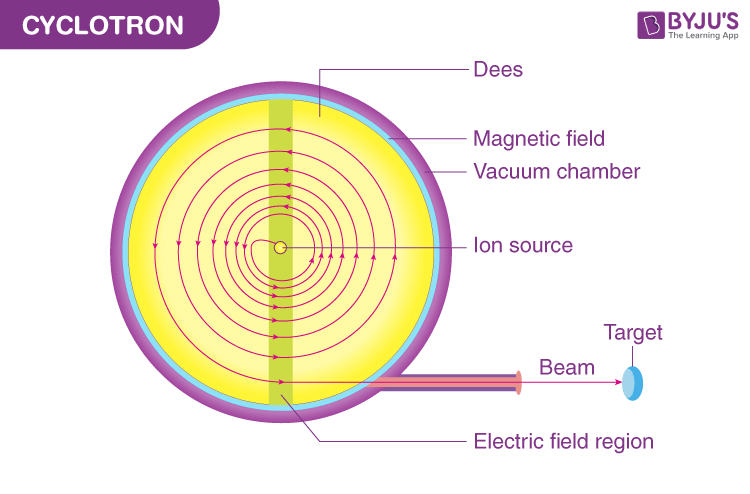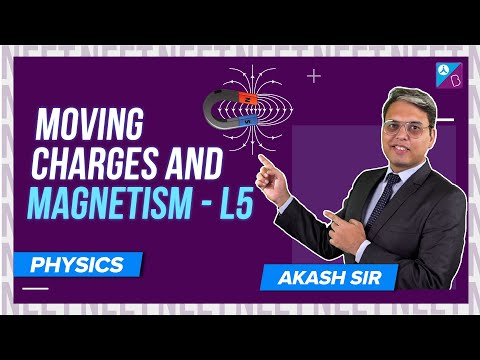# Cyclotron

## What Is a Cyclotron?

A cyclotron is a machine that accelerates charged particles or ions to high energies. It was invented to investigate the nuclear structure by E.O Lawrence and M.S Livingston in 1934. Both electric and magnetic fields are used in the cyclotron to increase the energy of the charged particles. As both the fields are perpendicular to each other, they are called cross fields.

In a cyclotron, charged particles accelerate outwards from the centre along a spiral path. These particles are held to a spiral trajectory by a static magnetic field and accelerated by a rapidly varying electric field.## Working Principle of Cyclotron

• A cyclotron accelerates a charged particle beam using a high frequency alternating voltage which is applied between two hollow “D”-shaped sheet metal electrodes known as the “dees” inside a vacuum chamber.
• The dees are placed face to face with a narrow gap between them, creating a cylindrical space within them for particles to move. Particles are injected into the centre of this space.
• Dees are located between the poles of electromagnet which applies a static magnetic field B perpendicular to the electrode plane.
• The magnetic field causes the path of the particle to bend in a circle due to the Lorentz force perpendicular to their direction of motion.
• An alternating voltage of several thousand volts is applied between the dees. The voltage creates an oscillating electric field in the gap between the dees that accelerates the particles.
• The frequency of the voltage is set so that particles make one circuit during a single cycle of the voltage. To achieve this condition, the frequency must be set to the particle’s cyclotron frequency.

### Expression for Cyclotron Frequency

$$\begin{array}{l}f=\frac{qB}{2\pi m}\end{array}$$

B is the magnetic field strength
q is the electric charge of the particle
m is the relativistic mass of the charged particle

### Expression for Particle Energy

The energy of the particles depends on the strength of the magnetic field and the diameter of the dees.

The centripetal force required to keep the particles in a curved path is given by the formula:

$$\begin{array}{l}F_c=\frac{mv^2}{r}\end{array}$$

The force is provided by the Lorentz’s force FB on the magnetic field B

$$\begin{array}{l}F_B=qvB\end{array}$$

Equating these equations, we get

$$\begin{array}{l}\frac{mv^2}{r}=qvB\end{array}$$

$$\begin{array}{l}v=\frac{qBR}{m}\end{array}$$

Hence, the output energy of the particles is given by the expression

$$\begin{array}{l}E=\frac{q^2B^2R^2}{2m}\end{array}$$

#### Cyclotron Solved Example:

A cyclotron has an oscillator frequency of 10 MHz. What should be the operating magnetic field for accelerating protons? Also, calculate the kinetic energy (in MeV) of the proton beam produced by the accelerator. (e = 1.60 × 10–19 C, mp = 1.67 × 10–27 kg, 1 MeV = 1.6 × 10–13 J)

Solution:

The oscillator frequency should be equal to the proton’s cyclotron frequency.

We use the following formula to find the strength of the magnetic field:

$$\begin{array}{l}B=\frac{2\pi mv}{q}\end{array}$$

Substituting the values in the above equation, we get

$$\begin{array}{l}B=\frac{6.3\times 1.67\times10^{-27} \times10^{7}}{1.6\times 10^{-19}}=0.66\,T\end{array}$$

The final velocity of the proton can be calculated using the following formula:

$$\begin{array}{l}v=r\times 2\pi v\end{array}$$

Substituting the values in the above equation, we get

$$\begin{array}{l}v=0.6\times 6.3\times 10^7=3.78\times 10^7\,m/s\end{array}$$

To find the kinetic energy of the proton, we use the following formula:

$$\begin{array}{l}E=\frac{1}{2}mv^2\end{array}$$

Substituting the values in the above equation, we get

$$\begin{array}{l}E=\frac{1.67\times 10^{-27}\times 14.3\times 10^{14}}{2\times 1.6\times 10^{-13}}=7\,MeV\end{array}$$

The kinetic energy of the proton beam produced by the accelerator is 7 MeV.

## Uses of Cyclotron

For several decades, these were the best sources of high-energy beams for nuclear physics experiments. However, these are still in use for this type of research.

### Treatment of Cancer:

Cyclotrons can be used in particle therapy to treat cancer, using the ion beams from cyclotrons to penetrate the body and kill tumours by radiation damage.## Are There Any Limitations to Cyclotron?

• Cyclotrons cannot accelerate electrons because electrons are of very small mass.
• A cyclotron cannot be used to accelerate neutral particles.
• It cannot accelerate positively charged particles with large mass due to the relativistic effect.

Stay tuned to BYJU’S to learn more physics concepts with the help of interactive video lessons.